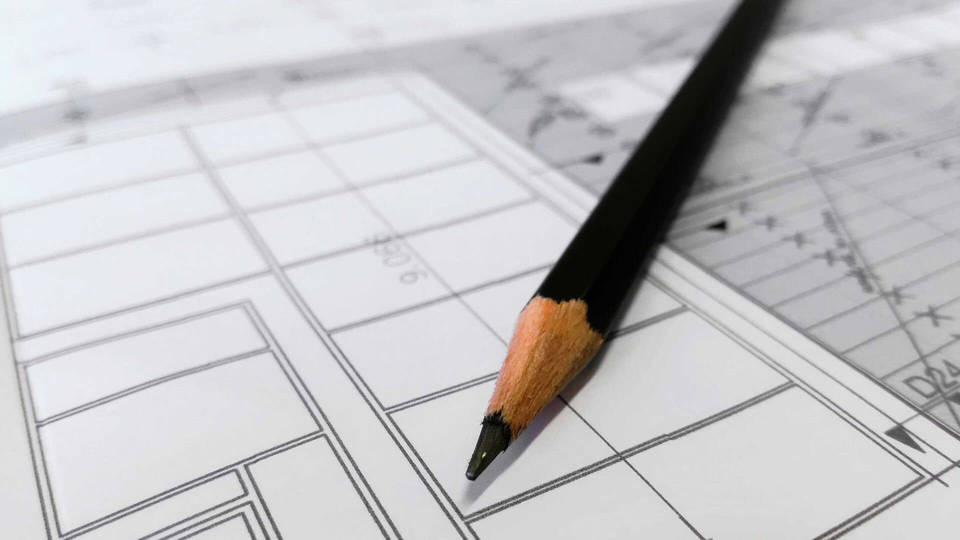Home buyers, sellers and real estate agents like to discuss most about price per square foot when comparing homes and estimating their value. It is an easy number to calculate (if you use our online calculators on squarefootagearea.com) and an easy concept to grasp. If you are going to use this metric in the home buying (or selling) process, you need to be aware of how to calculate it and how to make sure your measurements and calculations are accurate.

You need to question the accuracy of the data being presented, account for the uniqueness in each piece of real estate, and be sure that you are comparing very similar properties in the process as under or overestimating the true market value of a property can be devastating to your buying decision.

Who measures the square footage of a home?
Most square footage numbers are sourced from a previous listing, a recent appraisal, county tax records, or a floor plan originally provided by the builder. Of course, real estate agents and home owners might need to check-up on the figures themselves in case the builder’s plans have not been updated in recent years to the modifications and extensions made to the property.

Understanding the Square footage:
To understand how to calculate square footage we must first begin with the definition of area. An area is the size of a two-dimensional surface. The area of a square is the space contained within a set of lines. These lines should be measured in feet (ft) for square footage calculations and if needed, converted to inches (in), yards (yd), centimetres (cm), millimetres (mm) and metres (m).

Is the square footage accurate?
Square footage data is often littered with inaccuracies, so beware. Most notorious are older homes that may have been remodelled or expanded over the years. Records from the county tax assessor may be based on the original size of the home and fail to report the remodelling over the years. This seems ot be a common issue. Depending on your country of residence, there may not be a great mechanism for the government to keep accurate records of these changes, and data entry errors are common when transcribing from century-old records. So we have come up with a more reliable way to calculate the square footage of your property. Use our calculator below:
Square Footage Area Calculator

#### Formula:

Area of a Square= Side length x Side length
Answer = (Length x Width) square area
Abbreviations of unit area: ft2, in2, yd2, cm2, mm2, m2

Where do you need it in daily life?
Our Square Footage Calculator / SQFT calculator helps you calculate the area required for roofing shingles, landscaping for a garden, tiling, flooring space, carpeting and wallpaper. There is even a Siding Square Footage Calculator so you can estimate House Siding costs without any hassle.

What measurements do you need?
You need to know the length and the width of the area you wish to measure in either feet (ft), inches (in), yards (yd), centimetres (cm), millimetres (mm) or metres (m).

What can you calculate with this tool?
You can calculate the area in square feet, in square inches, square yards, square centimetres, square millimetres and square meters. Our calculator gives you the option of calculating the exact cost of materials. All you have to do is enter the price per unit area and voila, you have the total cost of materials in a single click!

Conversion factors:
To convert among square feet, square inches, square yards, square centimetres, square millimetres and square meters you can utilize the following conversion table.

 Square feet to yards multiply ft2 by 0.11111 to get yd2 Square feet to meters multiply ft2 by 0.092903 to get m2 Square yards to square feet multiply yd2 by 9 to get ft2 Square yards to square meters multiply yd2 by 0.836127 to get m2 Square meters to square feet multiply m2 by 10.7639 to get ft2 Square meters to square yards multiply m2 by 1.19599 to get yd2 Square meters to square millimetres multiply the m2 value by 1000000 to get mm2 Square meters to square centimetres multiply the m2 value by 10 000 to get cm2 Square centimetres to square metres multiply the cm2 value by 0.0001 to get mm2 Square centimetres to square millimetres multiply the cm2 value by 100 to get mm2 Square millimetres to square centimetres multiply the mm2 value by 0.000001 to get cm2 Square millimetres to square metres multiply the mm2 value by 1000000 to get m2

Expert real estate agents have clarified the process in the following explanation, “There is a specific methodology for measuring square footage, and it is relatively straightforward for rectangular homes with an easily-measured footprint. (Exterior measurements are most commonly used, and wall thicknesses are included in the calculations.) Many homes have difficult to measure spaces that can easily cause mistakes. For rooms with sloped ceilings, you need to account for the actual ‘usable’ space. Bay windows and circular floor plans may add challenging measurements. Open areas and staircase openings need to be deducted from the upper floor. You get the idea!

“Home building is imprecise by its nature. Architects create precise drawings that are translated in the field during the building of a home. Building materials and craftsmen are imperfect and may improvise and ‘shim’ their way to a close approximation of the architect’s drawing. These ‘as-built’ imperfections do materially impact the overall square footage, so the home may have deviations from the area measured in the original floor plan.”

So square footage area is an important tool which is useful to home owners and real estate agents alike.

Want us to cover a specific topic? Write to us.